﻿SURFACE CHEMISTRYSURFACE CHEMISTRY

SOLVED QUESTIONSChemistry 12th Class

Surface chemistry

Q1.

Adsorption is phenomenon is which a substance

a) Goes into the body of the other substance

(b) Remains close the other substance

(c) Accumulates on the surface of the other substance

(d) None of these

Solution.

Adsorption is a surface phenomenon. The phenomenon of attracting and retaining the molecules of a substance on the surface of another substance resulting into a higher concentration of the molecules on the surface is called adsorption.

Option ‘C’ is correct

Q2.

Adsorption is multilayered in the case of

(b) Chemisorption

(c) Both

(d) None of both

Solution:

Option ‘ A’ is correct

Q.3

Lyophobic colloids are

a) Reversible colloids (b) Irreversible colloids

(c) Protective colloids (d) Gum proteins

Solution: Lyophobic means – ‘’solvent-hating’’

Lyophobic colloids are not much hydrated and are easily precipitated and hence are not stable. These are irreversible in nature i-e once precipitated they do not form colloidal solution by simple mixing the two.

Option ‘B’ is correct

Q4.

Milk is a colloid in which

(a) A liquid is dispersed in liquid

(b) A solid is dispersed in liquid

(c) A gas is dispersed in liquid

(d) Some sugar is dispersed in water

Solution: Milk is a colloidal solution of oil in water type emulsion. Here liquid fat molecules (dispersed phase) are dissolved in water (dispersion medium).

Option ‘A ‘is correct.

Q5.

A colloidal system in which gas bubbles are dispersed in a liquid is known as

(a) Foam (b) Sol (c) Aerosol (d) Emulsion

Solution:

Colloidal solution in which dispersed phase is gas and dispersion medium is liquid is called ‘Foam’.

Colloidal solution in which dispersed phase is solid and dispersion medium is liquid is called ‘sol’

Colloidal solution in which dispersed phase is solid and dispersion medium is gas is called ‘Aerosol’

Colloidal solution in which dispersed phase is liquid and dispersion medium is liquid is called ‘emulsion’

Hence option ‘A’ is correct

Q6.

Dialysis is the process of separation of

(a) Suspended particles from colloids

(b) Suspended particles from crystalloids

(c) Colloidal particles from crystalloids

(d) Colloidal particles from gel

Solution: Dialysis is the purification process of colloidal solutions. The process of separating the particles of colloids from those of crystalloids by diffusion of a mixture through a suitable membrane is known as dialysis.

Option ‘C’ is correct

Q7.

Blood may be purified by

(a) Dialysis (b) Electro – osmosis

(c) Coagulation (d) Filtration

Solution: Blood is colloidal solution. The waste products in our blood can be separated from blood cells by dialysis. The dialysis membrane permits waste products to pass through whereas colloid-sized particles such as hemoglobin do not pass through the membrane.

Option “A” is correct

Q8

Enzymes are

(a) Substances made by chemists to activate washing powder

(b) Very active vegetable catalysts

(c) Catalysts found in organism

(d) Synthetic catalysts

Solution:

Enzymes are proteins produced by living systems and catalyse certain biological reactions. Enzymes are highly specific in nature.

Option “C” is correct.

Q9.

Which of the following statements about a catalyst is true

(a) It lowers the energy of activation

(b) The catalyst altered during the reaction is regenerated

(c) It does not alter the equilibrium

(d) All of these

Solution: A catalyst alters the reaction path by decreasing the activation energy of a reaction and hence the reaction becomes fast. Catalyst is involved in the reaction but is regenerated back at the end of the reaction i-e catalyst is not consumed during the reaction. It can’t alter the state of an equilibrium, only it speeds up the attainment of equilibrium.

Option ‘D’ is correct.

Q10.

Difference between colloids and crystalloids is of

(a) Particle composition

(b) Particle size

(c) Concentration

(d) Ionic character

Solution: Crystalloids are smaller in size than Colloids. Crystalloids can pass through the parchment membrane while as colloids can’t.

Option “B” is correct.

Q11.

The difference between a lyophilic and lyophobic colloid is in their

(a) Particle size (b) Behaviour towards dispersion medium

(c) Filtrability (d) None of these

Solution: lyophilic colloid is ‘Solvent loving’ and lyophobic colloid is ‘solvent hating’. Hence they differ in their behaviour towards dispersion medium.

Q12.

Which characteristic is true in respect of colloidal solutions?

(a) They always have two phases (b) They are only in liquid state

(c) They can’t be electrolysed (d) They are only hydrophilic

Solution: colloidal solution is heterogeneous in nature i-e it has two phases called dispersed phase and dispersion medium.

Option “A” is correct

Q13.

Which of the following statement is wrong for lyophobic sol

(a) Dispersed phase is heterogeneous in nature

(b) Can be easily coagulated by small addition of electrolyte

(c) Dispersed phase particles are poorly hydrated and colloid is stabilised due to charge on the colloidal particles

(d) Reversible in nature that is after coagulation can be easily set into colloidal form

Solution: lyophobic sol is irreversible that is after coagulation cannot form colloidal sol by simply mixing a dispersed phase and a dispersion medium. Lyophobic sol can be coagulated by adding a small amount of electrolyte because lyophobic sol is not much hydrated and hence is unstable.

Option “D’

Q14.

Following is the variation of physical adsorption with temperature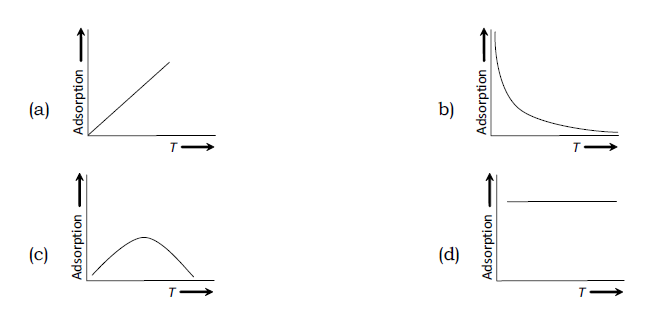Solution: physical adsorption decreases with increase in temperature. The adsorbed molecules gain energy and desorption takes place. As the temperature increase more molecules will be removed from the surface of absorbent.

Option”B”

Q15.

(a) P0 (b) P (c) Pn (d) P1/n

Solution: Freundlich adsorption isotherm is written as

X/m = KP1/n

P = pressure of the gas K= contant and ‘n’ is positive integer .

Hence Option “D”

Q16.

Which of the following is true in respect of the adsorption

(a) H must be negative (b) S must be negative

(c) G must be positive (d) H and S both are negative

Solution:

In an adsorption process H is negative means energy is released when adsorbate particle is adsorbed on the surface of adsorbent and entropy also decreases.

So both H and S are negative

Option “D”

Q17.

Which one of the following statement is not correct?

(b) The extent of adsorption depends on the pressure of the gas

(c) The extent of adsorption depends on the temperature

(d) The extent of adsorption has no upper limit

Solution: the extent of adsorption depends on

b)Pressure of a gas

c)Temperature

Extent of adsorption increase with the increase in pressure of a gas, however a point reaches when extent of adsorption does not change further with the increase in pressure- called saturation pressure.

Option “D”

Q18.

Which one is Freundlich’s equation

a)x/m=log K +1/n log P

b)x/m = exp(-KP)

c)x/m= KP2

d)log x/m = log K + 1/n log C

Solution: Freundlich adsorption isotherm is written as

X/m = KP1/n

P = pressure of the gas K= constant and ‘n’ is positive integer.

Taking log of both sides

Log x/m = log K + 1/n log P

For aqueous solution we use term concentration “C” rather than “P”

Log x/m = log K + 1/n log C

Q19.

For the adsorption of a gas on a solid, the plot of log (x/m) versus log P is linear with slope equal to

(a) K (b) log K (c) n (d) 1/n

Solution: Freundlich adsorption isotherm is written as

X/m = KP1/n

P = pressure of the gas K= constant and ‘n’ is positive integer.

Taking log of both sides

Log x/m = log K + 1/n log P

A graph between log x/m against Log P gives a straight line with slope equal to 1/n and ordinate intercept equal to Log K.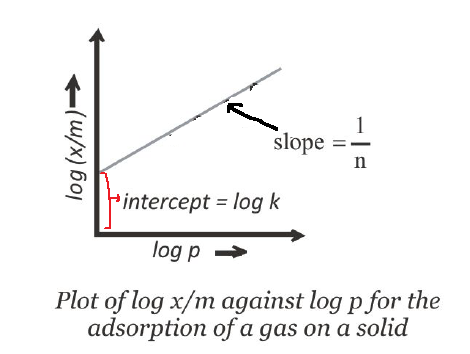Q20.

In a homogeneous catalysis

(a) The catalyst and the reactants should be gases

(b) The catalyst and the reactants should form a single phase

(c) Catalyst and the reactants are all solids

(d) The catalyst and the reactions are all liquids

Solution: In the homogeneous catalysis, the reaction mixture and the catalyst form a single phase.

If reactants and products are in gaseous phase, catalyst is also in the gas phase.

And if reactants and products are in liquid phase, catalyst is also in the liquid phase. E.g.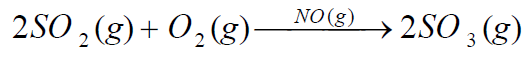Q21.

For the adsorption of solution on a solid x/m = KC1/n. Adsorption isotherm of log x/m and log C was found of the type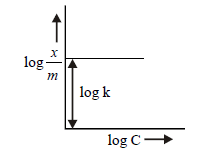This is when

(a)C=0

(b)C=1M

(c)C = constant

(d)C = 2M

Solution: Freundlich adsorption isotherm for solution is written as

X/m = KC1/n

C = Concentration of solution K= constant and ‘n’ is positive integer.

Taking log of both sides

Log x/m = log K + 1/n log C

When C= 1 M

1/n Log 1 = 0

Hence Log x/m = log K and slope of the graph will be zero

Hence option “B”

Q.22.

Colloidal particles carrying charge is best shown by

(a) Brownian movement (b) Tyndall effect

(c) Electrophoresis (d) Dialysis

Solution: The movement of colloidal particles under the influence of an electric field is called electrophoresis. The existence of a charge on a colloidal particle can be shown by the process of Electrophoresis.

Brownian movement is the Zig Zag movement of colloidal particles in a colloidal sol.

Tyndal effect is the scattering of light by the colloidal particles present in a colloidal solution.

Dialysis is a purification process of colloidal solution using a parchment membrane.

Hence option “C”

Q23.

Colloidal solution is not purified by

(a) Dialysis (b) Electro-dialysis (c) Electrophoresis (d) Ultrafiltration

Solution: Dialysis, Electro-dialysis, Ultrafiltration are purification methods for colloidal solutions while as Electrophoresis is a Coagulation process of colloidal solution. The movement of colloidal particles under the influence of an electric field is called electrophoresis.

Hence option “C”

Q24.

Peptizing agent is

(a) Always an electrolyte (b) Always a non - electrolyte

(c) Electrolyte or non - electrolyte (d) A lyophilic colloid

Solution: Peptization is process of passing of a precipitate into colloidal particles on adding suitable electrolyte. The electrolyte added is celled Peptizing agent.

Hence option “A”

Q25.

Lyophilic sols are more stable than lyophobic sols because

(a) The colloidal particles have positive charge

(b) The colloidal particles have no charge

(c) The colloidal particles are solvated

(d) There are strong electrostatic repulsions between the negatively charged colloidal particles

Solution: lyophilic colloid is ‘Solvent loving’ and lyophobic colloid is ‘solvent hating’. Lyophilic colloidal particles are highly hydrated hence more stable, while as lyophobic colloidal particles are not much hydrated.

Hence Option “c”

Q26.

Cleansing action of soap occurs because

(a) Oil and grease can be absorbed into the hydrophobic centres of soap micelles and washed away

(b) Oil and grease can be absorbed into the hydrophilic centres of soap micelles and washed away

(c) Oil and grease can be absorbed into both hydrophilic and hydrophobic centres but not washed away

(d) Cleansing action is not related to micelles

Solution: Soap molecule has a hydrophilic end and a long chain hydrophobic part.

Oil and grease being hydrophobic are absorbed into the centres of soap micelles and upon rubbing are washed away.

Hence option “A”

Q27.

Sky looks blue due to

(a)Dispersion effect (b) Reflection (c) Transmission (d) Scattering

Solution: Atmosphere is a colloidal system as it contains tiny dust particles in atmospheric gases. And due to tyndal effect the blue light coming from the sun gets scattered. The amount of light scattered is inversely proportional to the fourth power of wavelength. It follows that blue light is scattered more than red light by a factor of about 10.

Hence option “D”

Q.28.

Ferric hydroxide sol is positively charged colloid. The coagulating power of NO3- , SO42- and PO43- ions would be in the order

(a) NO3- > SO42- > PO43-

(b) SO42- > NO3- > PO43-

(c) PO43- > SO42- > NO3-

(d) NO3- = SO42- = PO43-

Solution: The number of millimoles of an electrolyte which is required to cause the coagulation of a sol is known as coagulation value.

Coagulation value is inversely proportional to coagulation power.

For a positively charged sol, the coagulation power of an anions is directly proportional to the negative charge on anion.

Hence option “C”

Q29.

Which one of the sols acts as protective colloid

(a) As2S3 (b) Gelatin (c) Au (d) Fe(OH)3

Solution: the stability of the lyophobic colloidal sols can be increased by adding a small amount of lyophilic colloids. This process is called protection and the lyophilic colloid is termed as protective colloid.

Among the above mentioned colloids, Gelatin is lyophilic colloid while as others are lyophobic.

Hence option “B”

Q30.

Maximum coagulation power is in

(a) Na+ (b) Ba2+ (c) Al3+ (d) Sn4+

Solution: the coagulation power of a cation is directly proportional to the positive charge on cation

Hence option “D”

Q31.

In the shown figure, n=Xm where m is the mass of adsorbent and X that of adsorbate. Which of the following option is correct?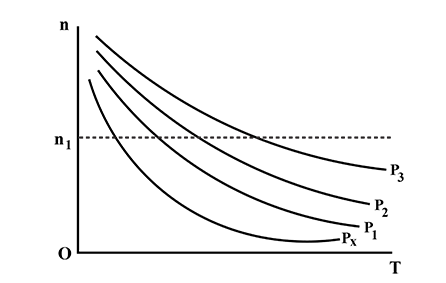(a) P1 > P2 > P3

(b) P3 > P2 > P1

(c) P1 > P2 = P3

(d) P1 = P2 = P3

Solution: Amount of adsorption decreases with increase in temperature and the higher pressure means more adsorption because as the pressure increases amount of adsorption will also increase.

Hence option “B”

Q32.

The mole of gases NH3, CO2 and H2 adsorbed by 1 g charcoal at 300 K and 1 atm pressure shows the order:

(a)NH3 < CO2 < H2

(b)NH3 = CO2 =H2

(c)NH3 > CO2 > H2

(d)NH3 > CO2 = H2

Solution: Extent of adsorption depends on the nature of a gas. Higher the critical temperature of a gas more will be the extent of adsorption. The critical temperature of NH3 , CO2 and H2 decreases in the order of

NH3 > CO2 > H2

Hence option “C”

Q33.

Coagulation or demulsification can be done by some of the methods given below

I: By addition of substance which would destroy the emulsifier

II: By addition of an electrolyte which would destroy the charge

III: By heating, freezing and centrifuging

Select correct methods

(a) I, II (b) I, II, III (c) II only (d) III only

Solution: Coagulation occurs by destroying the emulsifier. Emulsifiers stabilize the colloidal particles. Destroying charge on the colloidal particles also causes the coagulation.

Heating, freezing and centrifugation also destabilize the colloidal solution.

Hence Option “B”

Q34.

There are certain properties related to physical adsorption

I : Reversible

II : Formation of unimolecular layer

III : Low heat of adsorption

IV : Occurs at low temperature and decreases with increasing temperature

Which of the above properties are for physical adsorption

(a) I, II, III (b) I, III, IV (c) II, III, IV (d) I, III

Solution: physical adsorption is reversible, multi-molecular layers are formed and as the forces operating in these cases are weak van-der Waals forces, hence heats of adsorption are low. The amount of adsorption decreases with increase in temperature.

Hence option “B”

Q35.

Following are the events taking place to explain adsorption theory

I : Desorption

II : Diffusion of the reactants along the surface

III : Adsorption of the reactants

IV : Formation of the activated surface complex

These events are taking place in the following order

(a) I, II, III, IV (b) III, II, IV, I (c) III, IV, I, II (d) IV, III, II, I

Solution: The adsorption (as per adsorption theory) is believed to take place in the following steps-

2.Then the reactants are diffused towards the surface where they react

3.Now the activated surface complex is formed.

4.The products formed on the surface are desorbed (desorption).

Hence option ”B”

Q36.

Chemisorption

(a) Increases with temperature (b) Decreases with temperature

(c) Remains unaffected by change of temperature

(d) First increases then decreases with temperature

Solution: Chemisorption first increases with increase in temperature and then decrease with increase in temperature. The initial increase is because of the fact that heat supplied acts as activation energy required in chemisorption. At higher temperature it behaves like a physical adsorption.

Hence option”D”

Q37.

Which of the following is not a characteristic of chemisorption

(a) H is of the order of 400 kJ (b) Adsorption is irreversible

Hence option “C”

Q38 . Adsorption of gases on solid surface is generally exothermic because

(a) Enthalpy is positive (b) entropy decreases

(c) Entropy increases (d) free energy increases

Solution: When the gas is adsorbed on surface, the freedom of movement of gaseous molecules becomes restricted. This causes decrease in the entropy of the gas after adsorption, i.e., S becomes negative. Hence, S becomes negative.

Hence option “B”

Q39.

As2S3 sol has a negative charge. Capacity to precipitate it is highest in

(a) AlCl3 (b) Na3PO4 (c) CaCl2 (d) K2SO4

Solution: For a negatively charged sol, the coagulation power of a salt is directly proportional to the positive charge on cation.

Al3+ > Ca2+ > Na+

Hence Option ”A”

Q40.

When saturation is attained in terms of adsorption, variation of x/m and C (concentration) is given by the portion of the isotherm

(a) OA (b) OB (c) AB (d) BC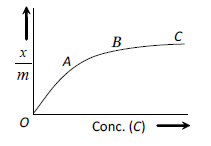Solution: when saturation is reached amount of adsorption (x/m) does not change with increase in concentration and hence slope of the graph is almost zero.

Hence option “D”